## Ostrowski's Theorem

Letbe a Matrix with Positive Coefficients andbe the Positive Eigenvalue in the Frobenius Theorem, then the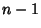Eigenvalues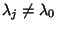satisfy the Inequalitywhere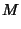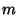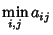and, 2, ...,.